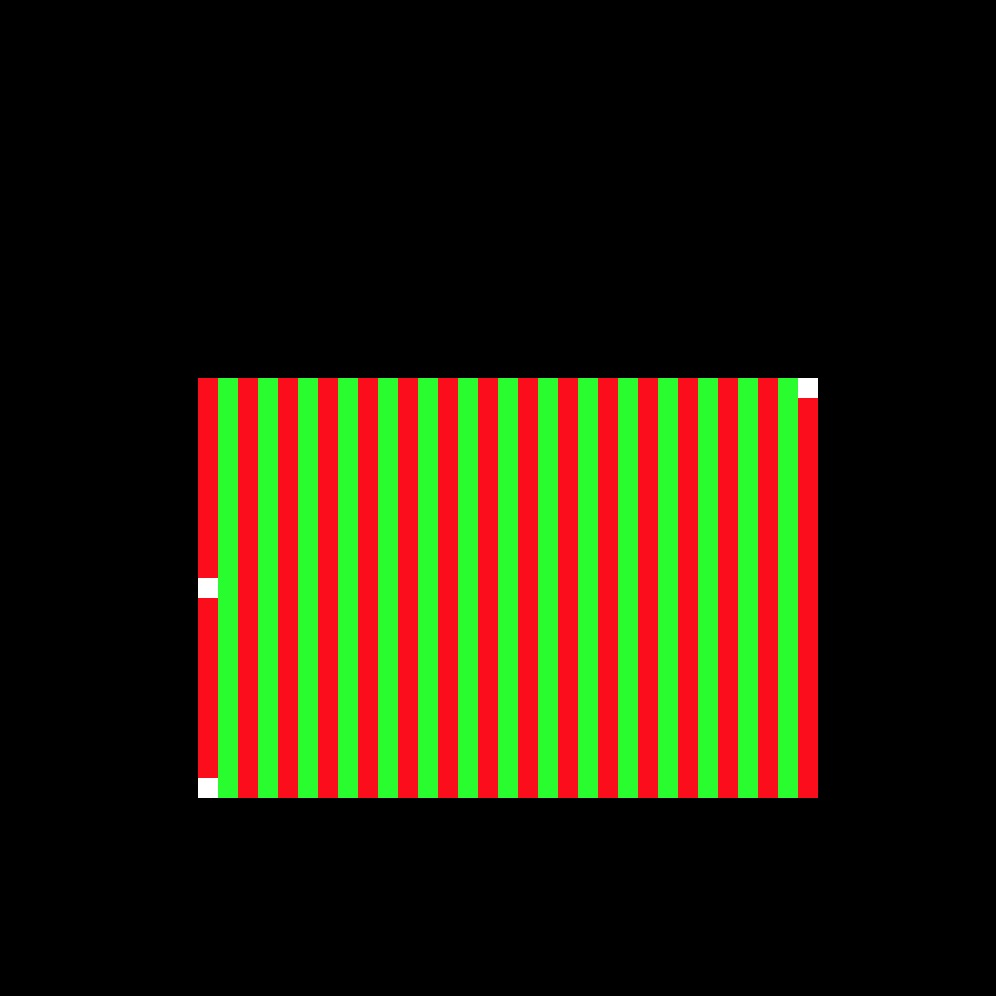# Lab 1 - Rasterizer: Bounding Box

## Overview and Context

For Assignment 1, we will be writing a program to render (draw) a polygonal mesh of triangles as an image via software rasterization (i.e. the code will be entirely written in C/C++ with no graphic libraries. The software will render a static scene). In general the required steps for the program will be:

• Convert triangles to window coordinates.
• Rasterize each triangle (using barycentric coordinates for linear interpolations and in-triangle test).
• Write color values per pixel (using a z-buffer test to resolve depth).

We will discuss the window coordinate transform, barycentric coordinates and z-buffers in the next few lectures.

## Goals for This Lab

For this lab, we will just be playing with the provided code (`main.cpp`) to build up functionality that will be useful in your rasterizer.

• Read in three vertices which represent a triangle (see below for details).
• Color all the pixels in the bounding box the color of your choice.
• Write out those pixels as an image (base code provided).

Starting with the provided code, make the list of command line arguments take the following options.

• Output filename (e.g., foo.png)
• Image width (e.g., 512)
• Image height (e.g., 512)
• Vertex 1 x-coord (e.g., 100)
• Vertex 1 y-coord (e.g., 100)
• Vertex 2 x-coord
• Vertex 2 y-coord
• Vertex 3 x-coord
• Vertex 3 y-coord

For this lab only, we will assume the triangle’s vertices are in “window coordinates.” In other words, the data values are integer values that range from `0` to the value of `width-1` of the window/image (or `0` to the `height-1` value of the window/image). You may choose to have the vertices be 2D (although for the Assignment 1, they will need to be 3D). You will need to design a data structure to represent the triangle and its vertices. Draw the three vertices into the image, using three calls to `image->setPixel()`.

The bounding box of the triangle is the box with the extents that will exactly bound that triangle. You will need to design a data structure to represent the bounding box. At minimum such a structure needs `xmin`, `xmax`, `ymin`, `ymax`. Using the provided image code, modify the color of all the pixels in the bounding box to be a color of your choice (which is different then the background).

You can also try a pattern based on the row and/or column. The three vertices and the bounding box should look something like this:Write out the modified image and confirm your code is working as expected. Be sure to try various test cases with vertex positions in various orders. Make sure the bounding box completely covers the three vertices.

Generated on Thu Jan 9 11:47:32 CST 2020